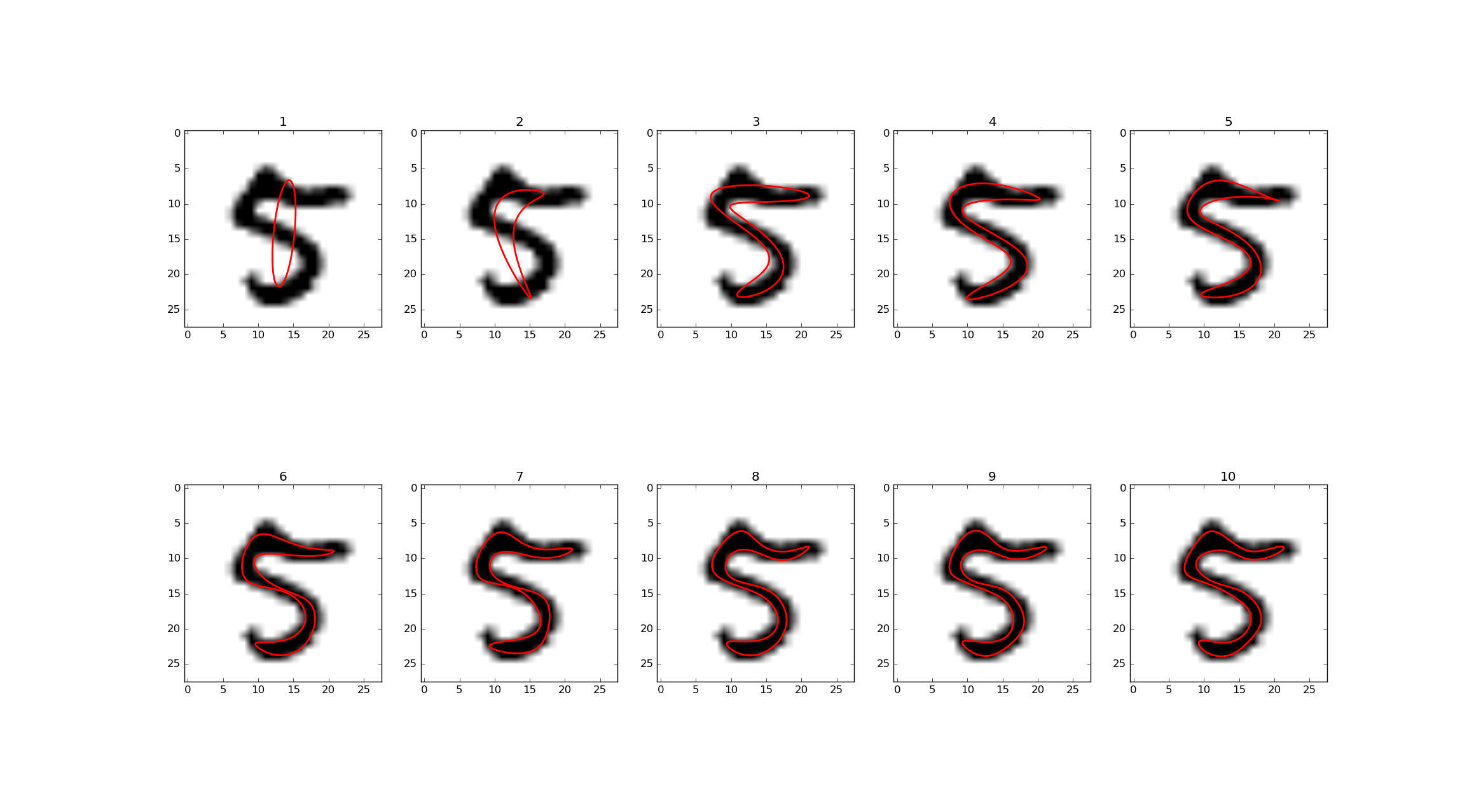# PyEFD¶An Python/NumPy implementation of a method for approximating a contour with a Fourier series, as described in .EFD representations of an MNIST  digit. Shows progressive improvement of approximation by order of Fourier series.

## Installation¶

$pip install pyefd  ## Usage¶ Given a closed contour of a shape, generated by e.g. scikit-image or OpenCV, this package can fit a Fourier series approximating the shape of the contour. ### General usage examples¶ This section describes the general usage patterns of pyefd. from pyefd import elliptic_fourier_descriptors coeffs = elliptic_fourier_descriptors(contour, order=10)  The coefficients returned are the $$a_n$$, $$b_n$$, $$c_n$$ and $$d_n$$ of the following Fourier series representation of the shape. The coefficients returned are by default normalized so that they are rotation and size-invariant. This can be overridden by calling: from pyefd import elliptic_fourier_descriptors coeffs = elliptic_fourier_descriptors(contour, order=10, normalize=False)  Normalization can also be done afterwards: from pyefd import normalize_efd coeffs = normalize_efd(coeffs)  ### OpenCV example¶ If you are using OpenCV to generate contours, this example shows how to connect it to pyefd. import cv2 import numpy from pyefd import elliptic_fourier_descriptors # Find the contours of a binary image using OpenCV. contours, hierarchy = cv2.findContours( im, cv2.RETR_TREE, cv2.CHAIN_APPROX_SIMPLE) # Iterate through all contours found and store each contour's # elliptical Fourier descriptor's coefficients. coeffs = [] for cnt in contours: # Find the coefficients of all contours coeffs.append(elliptic_fourier_descriptors( numpy.squeeze(cnt), order=10))  ### Using EFD as features¶ To use EFD as features, one can write a small wrapper function: def efd_feature(contour): coeffs = elliptic_fourier_descriptors( contour, order=10, normalize=True) return coeffs.flatten()[3:]  If the coefficients are normalized, then coeffs[0, 0] = 1.0, coeffs[0, 1] = 0.0 and coeffs[0, 2] = 0.0, so they can be disregarded when using the elliptic Fourier descriptors as features. See  for more technical details. ## Testing¶ Run tests with: $ python setup.py test


or with Pytest:

\$ py.test tests.py


The tests includes a single image from the MNIST dataset of handwritten digits () as a contour to use for testing.

## API¶

A Python implementation of the method described in  and  for calculating Fourier coefficients for characterizing closed contours.

### References¶

  (1, 2, 3) F. P. Kuhl and C. R. Giardina, “Elliptic Fourier Features of a Closed Contour,” Computer Vision, Graphics and Image Processing, Vol. 18, pp. 236-258, 1982.
  (1, 2, 3) Oivind Due Trier, Anil K. Jain and Torfinn Taxt, “Feature Extraction Methods for Character Recognition - A Survey”, Pattern Recognition Vol. 29, No.4, pp. 641-662, 1996

Created by hbldh <henrik.blidh@nedomkull.com> on 2016-01-30.

pyefd.calculate_dc_coefficients(contour)[source]

Calculate the $$A_0$$ and $$C_0$$ coefficients of the elliptic Fourier series.

Parameters: contour (numpy.ndarray) – A contour array of size [M x 2]. The $$A_0$$ and $$C_0$$ coefficients. tuple
pyefd.elliptic_fourier_descriptors(contour, order=10, normalize=False)[source]

Calculate elliptical Fourier descriptors for a contour.

Parameters: contour (numpy.ndarray) – A contour array of size [M x 2]. order (int) – The order of Fourier coefficients to calculate. normalize (bool) – If the coefficients should be normalized; see references for details. A [order x 4] array of Fourier coefficients. numpy.ndarray
pyefd.normalize_efd(coeffs, size_invariant=True)[source]

Normalizes an array of Fourier coefficients.

See  and  for details.

Parameters: coeffs (numpy.ndarray) – A [n x 4] Fourier coefficient array. size_invariant (bool) – If size invariance normalizing should be done as well. Default is True. The normalized [n x 4] Fourier coefficient array. numpy.ndarray
pyefd.plot_efd(coeffs, locus=(0.0, 0.0), image=None, contour=None, n=300)[source]

Plot a [2 x (N / 2)] grid of successive truncations of the series.

Note

Requires matplotlib!

Parameters: coeffs (numpy.ndarray) – [N x 4] Fourier coefficient array. tuple or numpy.ndarray locus (list,) – The $$A_0$$ and $$C_0$$ elliptic locus in  and . n (int) – Number of points to use for plotting of Fourier series.